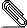# [NTG-context] Reflections the interplay between units and digits

Morning all…

I think that there are several issues in the interplay of the \units and
\digits command that could be a bit better (Sorry Hans…all meant in the best
possible way).digits_trial.pdf

So here is a complete example and the pdf I get, after some experimentation.
With kludges of my own. I'd guess there are several people using ConTEXt for
the sciences, so this may help some, or some may set me on a better path…

___

\starttext
\setdigitmode 6

{\em digit exponent powers - don't work}

\digits{20e-12}

\digits{-123.222,00e-12} % this one gives nothing at all

But it seems it should \emdash\ ConTEXt magazine3 May 2003, from which the
following non-working examples come

\digits 123.222,00^10 % this one gives nothing at all

\digits 123.222,00e10 % this one gives nothing at all

\digits /123.222,00e-12 % this one gives nothing at all

\digits -123.222,00e-12 % this one gives nothing at all

\digits +123.222,00e-12 % this one gives nothing at all

{\em spacing and decimal points}

\digits 12,345,000.90

Has the same effect as this hard-coded beasty.

12~345~000.78

Digits without exponents seem to work....

\digits 1230.92

\digits{12,460,800.89}

{\em Now a little challenge - write out the speed of light, with units. So to
combine units with digits}

\unit{299792458 meter inverse second} % en-route, but I'd like every three
digits separated by a non-breaking space

So try the digits command - two possibilities

%\digit {\unit{299792458 meter inverse second}} % digits nests units - well the
interpreter rejected this one

%\unit{\digit{299792458} meter inverse second} % units nests digits - and this
one.

Conclusion digits and units don't play nicely together...unless someone has a
nice solution

Maybe a bit hard coding - fugly, but maybe...

\unit{299~792~458 meter inverse second} % fails to interpret units, so no good

299~792~\unit{458 meter inverse second} % looks OK, but inelegant

Now for trying to write in standard form..first the inelegant form, but giving
a passable result

Speed = 3 \times\ \unit{10^8 meter inverse second}

But I'd guess this will not be inside a tex box, so the layout might be
dodgy... numbers and units should not be split over two lines...

A much more elegant solution would be:

Speed = \unit{3e8 meter inverse second} % the documentation seems to support
this, but not the practice...

This one would be cleanly coded, if only the exponentiation worked...

{\em spread of a physical quantity}
One more, whilst I'm here. People will want to indicate a spread in a physical
quantity, so:

\unit{400\endash 700 THz}   % inserts an unwanted space after the 400: ( 400
–700 THz). (cf 11\endash 14 , which 'behaves' as expected

You can again kludge, by

400\endash \unit{700 THz}

But elegant coding it ain't \ldots and again probably no bounding tex box, so
may get split over two lines.

\stoptext

___

KR
Ian
___________________________________________________________________________________
___________________________________________________________________________________